For what value of unknown resistance X, the potential difference between B and D will be zero in the circuit shown in the figure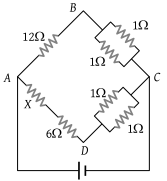(1) 4 Ω

(2) 6 Ω

(3) 2 Ω

(4) 5 Ω

Concept Questions :-

Combination of resistors
High Yielding Test Series + Question Bank - NEET 2020

Difficulty Level:

Which arrangement of four identical resistances should be used to draw maximum energy from a cell of voltage V

(1)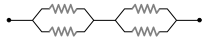(2)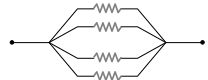(3)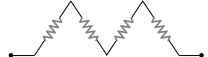(4)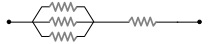Concept Questions :-

Combination of resistors
High Yielding Test Series + Question Bank - NEET 2020

Difficulty Level:

An unknown resistance R1 is connected in series with a resistance of 10 Ω. This combinations is connected to one gap of a metre bridge while a resistance R2 is connected in the other gap. The balance point is at 50 cm. Now, when the 10 Ω resistance is removed the balance point shifts to 40 cm. The value of R1 is (in ohm

(1) 60

(2) 40

(3) 20

(4) 10

Concept Questions :-

Combination of resistors
High Yielding Test Series + Question Bank - NEET 2020

Difficulty Level:

A wire has a resistance of 6 Ω. It is cut into two parts and both half values are connected in parallel. The new resistance is :

(1) 12 Ω

(2) 1.5 Ω

(3) 3 Ω

(4) 6 Ω

Concept Questions :-

Combination of resistors
High Yielding Test Series + Question Bank - NEET 2020

Difficulty Level:

Six equal resistances are connected between points P, Q and R as shown in the figure. Then the net resistance will be maximum between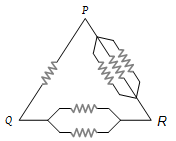(1) P and Q

(2) Q and R

(3) P and R

(4) Any two points

Concept Questions :-

Combination of resistors
High Yielding Test Series + Question Bank - NEET 2020

Difficulty Level:

The total current supplied to the circuit by the battery is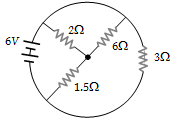(1) 1 A

(2) 2 A

(3) 4 A

(4) 6 A

Concept Questions :-

Combination of resistors
High Yielding Test Series + Question Bank - NEET 2020

Difficulty Level:

An electric current is passed through a circuit containing two wires of the same material, connected in parallel. If the lengths and radii of the wires are in the ratio of 4/3 and 2/3, then the ratio of the currents passing through the wire will be

(1) 3

(2) 1/3

(3) 8/9

(4) 2

Concept Questions :-

Combination of resistors
High Yielding Test Series + Question Bank - NEET 2020

Difficulty Level:

In circuit shown below, the resistances are given in ohms and the battery is assumed ideal with emf equal to 3 volt. The voltage across the resistance R4 is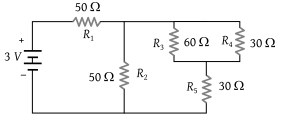(1) 0.4 V

(2) 0.6 V

(3) 1.2 V

(4) 1.5 V

Concept Questions :-

Combination of resistors
High Yielding Test Series + Question Bank - NEET 2020

Difficulty Level:

If you are provided three resistances 2 Ω, 3 Ω and 6 Ω. How will you connect them so as to obtain the equivalent resistance of 4 Ω

(1)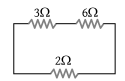(2)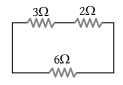(3)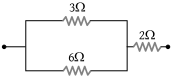(4) None of these

Concept Questions :-

Combination of resistors
High Yielding Test Series + Question Bank - NEET 2020

Difficulty Level:

The equivalent resistance and potential difference between A and B for the circuit is respectively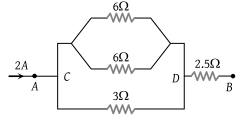(1) 4 Ω, 8 V

(2) 8 Ω, 4 V

(3) 2 Ω, 2 V

(4) 16 Ω, 8 V

Concept Questions :-

Combination of resistors